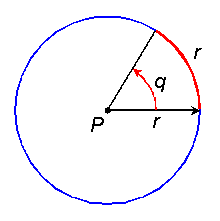WhatIs.com.com

By Margaret Rouse

The radian is the Standard International (SI) unit of plane angular measure. There are 2 pi, or approximately 6.28318, radians in a complete circle. Thus, one radian is about 57.296 angular degrees.

The term radian arises from the fact that the length of a circular arc, corresponding to an angle of one radian, is equal to the radius of the arc. This is shown in the illustration. Point P represents the center of the circle. The angle q, representing one radian, is such that the length of the subtended circular arc is equal to the radius, r, of the circle.The radian is used by mathematicians, physicists, and engineers. It arises in natural phenomena and in equations which, unlike the angular degree, were invented for human convenience.

Also see Standard International (SI) System of Units.

21 Sep 2005